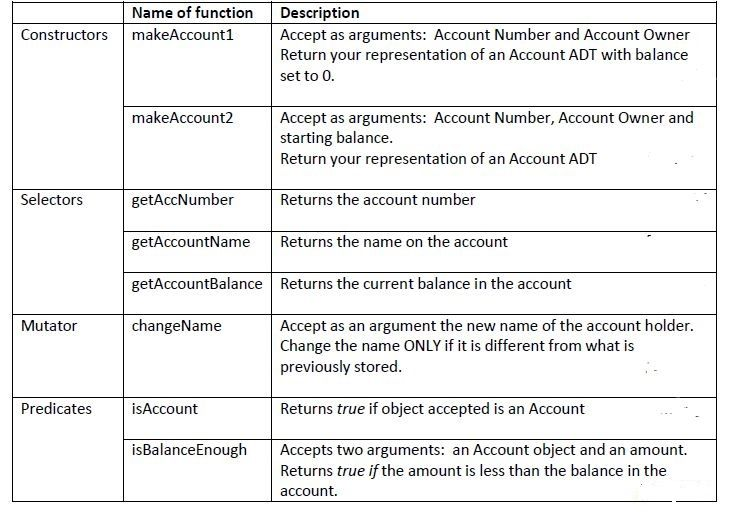Bank Account in PythonPosted by Samath4148 April 23, 2015

Decide on a way to represent a tagged Abstract Data Type called Bank Account. The data stored on a Bank Account are:
a) Account Number
b) Account Owner
c) Balance```def makeAccount1(accNum, accOwn):
return ['bAccount', accNum,accOwn,0]

def makeAccount2(accNum, accOwn, sBalance):
return ['bAccount', accNum,accOwn,sBalance]

def getAccNumber(acc):
return acc

def getAccountName(acc):
return acc

def getAccountBalance(acc):
return acc

def changeName(name, acc):
if(name != acc):
acc = name

def isAccount(acc):
if acc == "bAccount":
return True
else:
return False

def isBalanceEnough(acc,amt):
if(amt<acc):
return True
else:
return False```

Write functions to do the following:
a) Withdraw an amount from a given account, if sufficient money is available.

```def withdraw(acc, amt):
if(isBalanceEnough(acc,amt)):
acc-=amt```

b) Deposit an amount into a given account.

```def deposit(acc, amt):
acc+=amt```

c) Transfer money from a valid account to another valid account. Transference should ONLY be done if sufficient money is in the account.

```def transfer(fromAcc, toAcc, amt):
if(isAccount(fromAcc) and isAccount(toAcc)):
if(isBalanceEnough(fromAcc,amt)):
withdraw(fromAcc, amt)
deposit(toAcc, amt)
```

This question focuses on collections of Bank Accounts:
a) Choose to represent your collection of bank Account as a Stack or Queue.

```def stackAccount():
return ('stack',[])```

b) Write the relevant methods needed to (correct naming of your methods is extremely important):
i. Add an Account to the collection

```def addAccount(stack_i,acc):
stack_i.insert(0,acc)```

ii. Remove an Account from the collection

```def removeAccount(stack_i):
stack_i.pop(0)```

iii. Check what account is at the front or top

```def top(stk):
return stk```

iv. Check if the collection is empty

```def is_empty(stack_i):
if(stack_i==[]):
return True```

v. Output all account information (Formatting of output is important)

```def outputAccountFromStack(stk):
for a in stk:
print("Account Number: "+getAccNumber(a)+" Name: "+getAccountName(a)+" Balance: "+str(getAccountBalance(a)))```

5. Write a main method to do the following:
a) Accept account information and add your collection. (NB: Use a loop and ensure you allow ONLY valid accounts to the collection )
b) Charge a balance deduction fee of \$500, for accounts with less than \$2000 balance.
c) Charge a general 1% bank charges fee to ALL accounts.

```def main():

accountGroup = stackAccount()

while(True):
accNum = input("Enter account number: ")
accName = input("Enter account name: ")
accBalance = int(input("Enter account balance: "))

myAccount = makeAccount2(accNum, accName, accBalance)

if(isAccount(myAccount)):
else:
print("Invalid Account... Try Again")
continue

option = input("Enter Y to Add more Account N to Quit: ")
if(option == 'Y' or option == 'y'):
continue
elif(option == 'N' or option == 'n'):
break

for acc in accountGroup:
abal = getAccountBalance(acc)
if(abal < 2000):
withdraw(acc, 500)

for acc in accountGroup:
abal = getAccountBalance(acc)
charge = 0.01 * abal
withdraw(acc, charge)

outputAccountFromStack(accountGroup)```sherry October 23, 2015 samath i have a project of banking systen in python . can u make a project